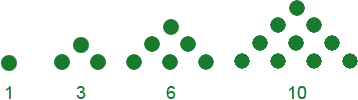SEARCH HOMEMath Central Quandaries & QueriesSubject: Finding the Nth term Name: Lisa Who are you: Student Hi, I am really stuck on this question, and I can't find the answer anywhere: HELP! What is the nth term for the sequence, '1, 3, 6, 10...' and could you say how you got the anwser. Thanks a lot, LisaHi Lisa. Whenever I get these kinds of problems, one of my first strategies is to look at the differences between the terms and/or the ratios between them to see if there is a pattern.

Your sequence is 1, 3, 6, 10, ... and the differences are 2, 3, 4, ... so actually each term is the sum of all the counting numbers up to the term number. So the fifth term in the sequence would be the sum of all the numbers up to 5: 1+2+3+4+5 = 15. This means the nth term is the sum of all the numbers up to n: 1+2+...+(n-1)+n.

Stephen La Rocque.>

Lisa,

There is a compact way to write 1+2+...+(n-1)+n, do you know what it is?

This is a really neat sequence of numbers, they are called triangular numbers.PennyMath Central is supported by the University of Regina and The Pacific Institute for the Mathematical Sciences.